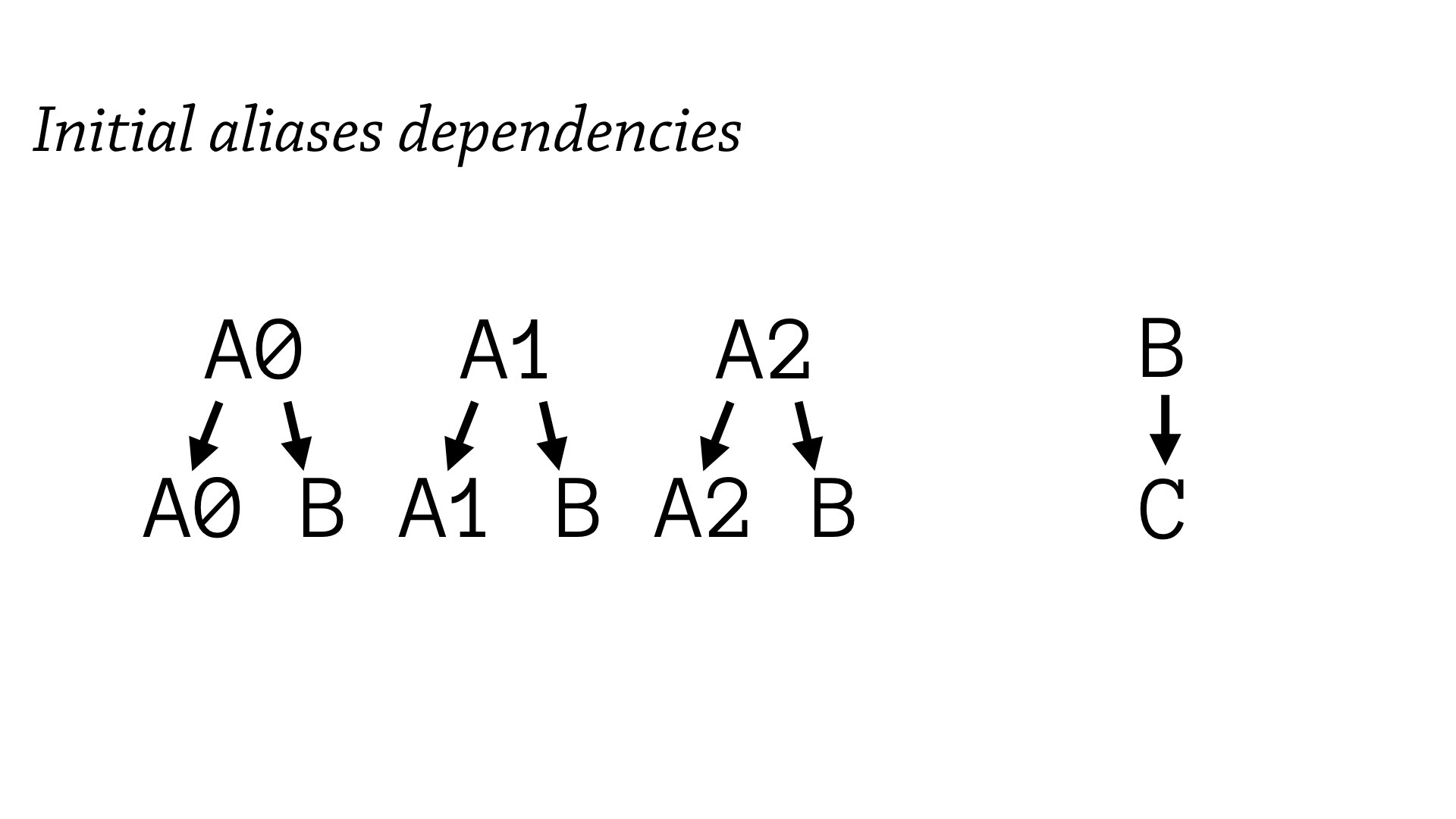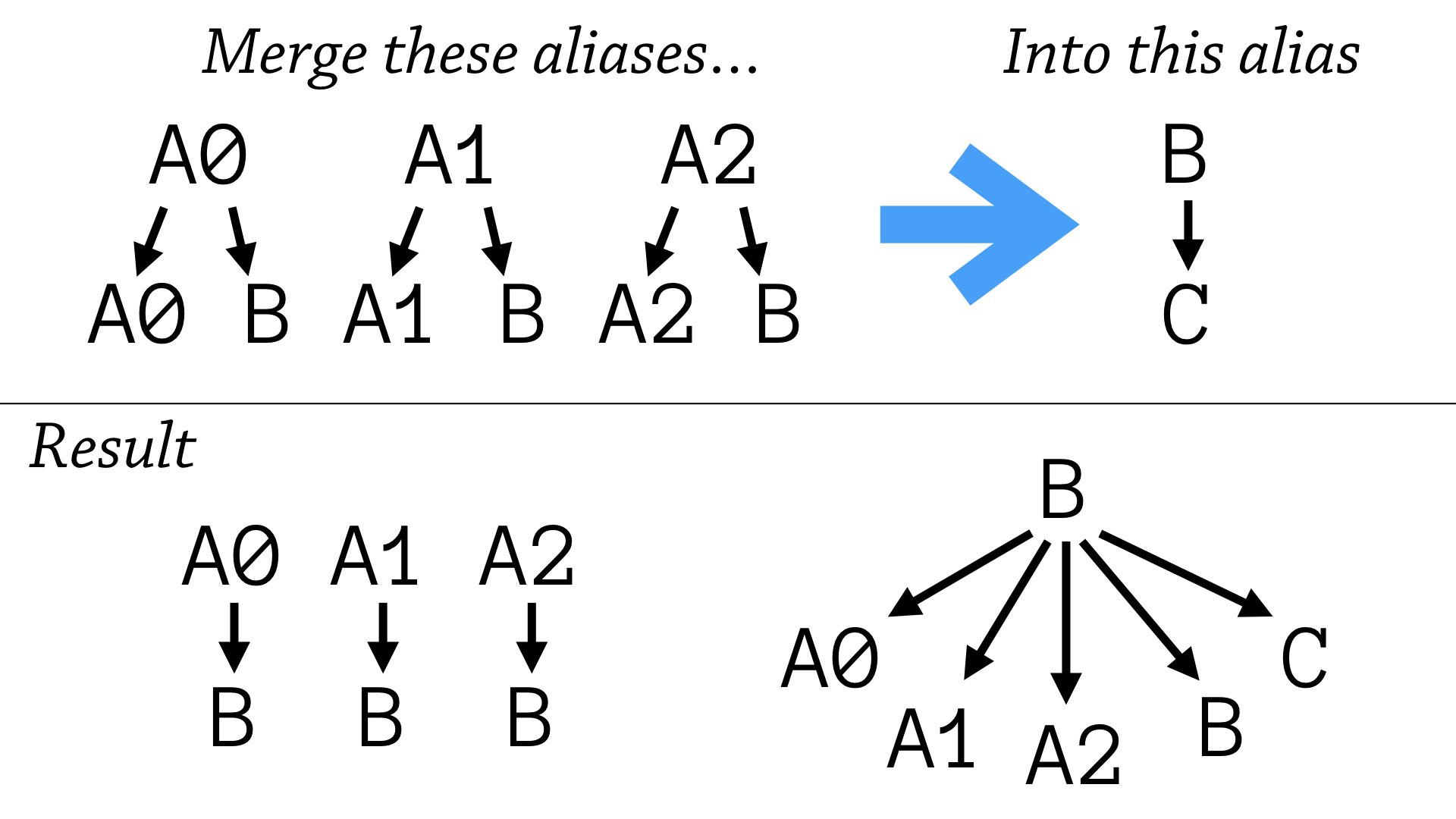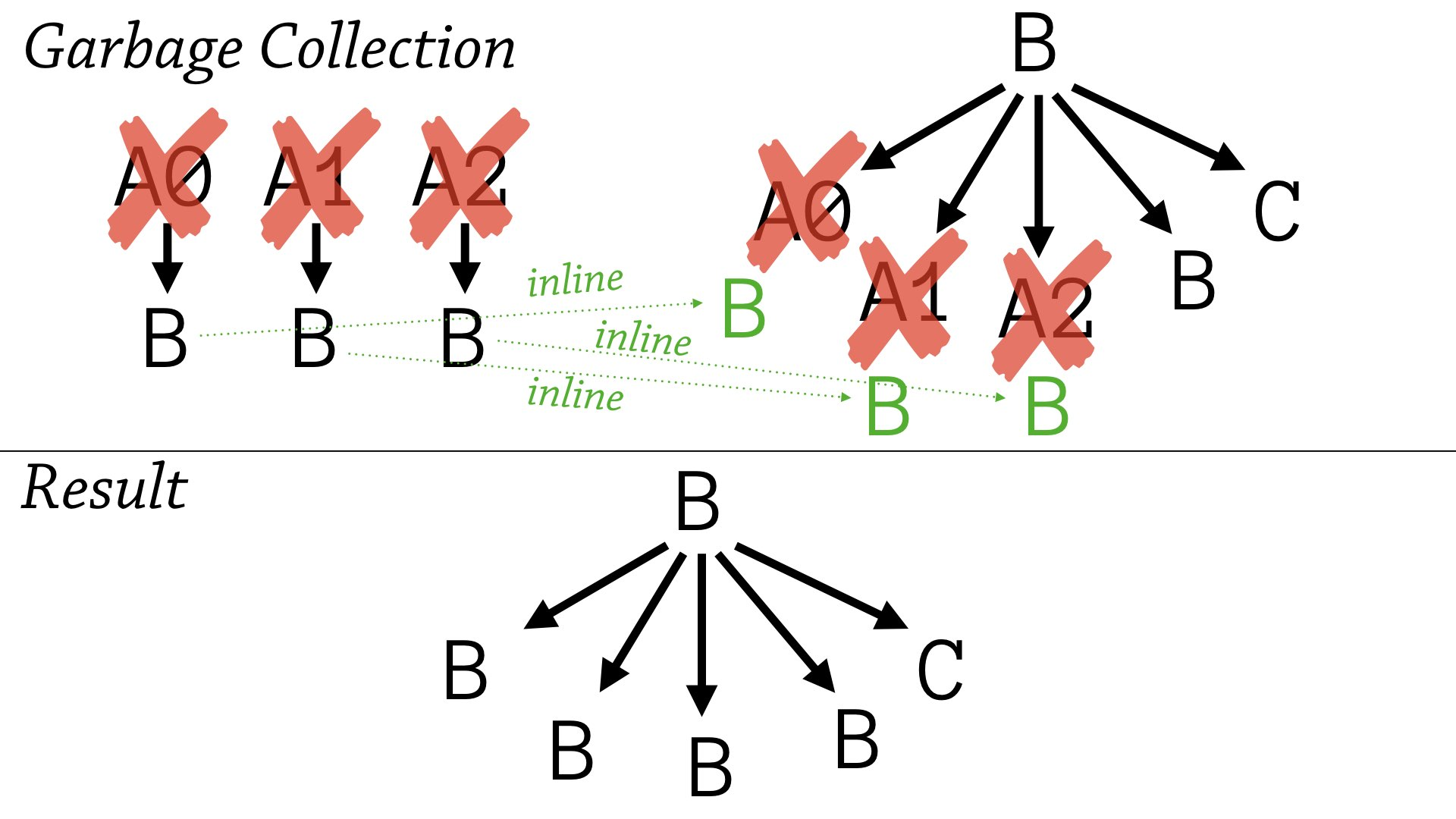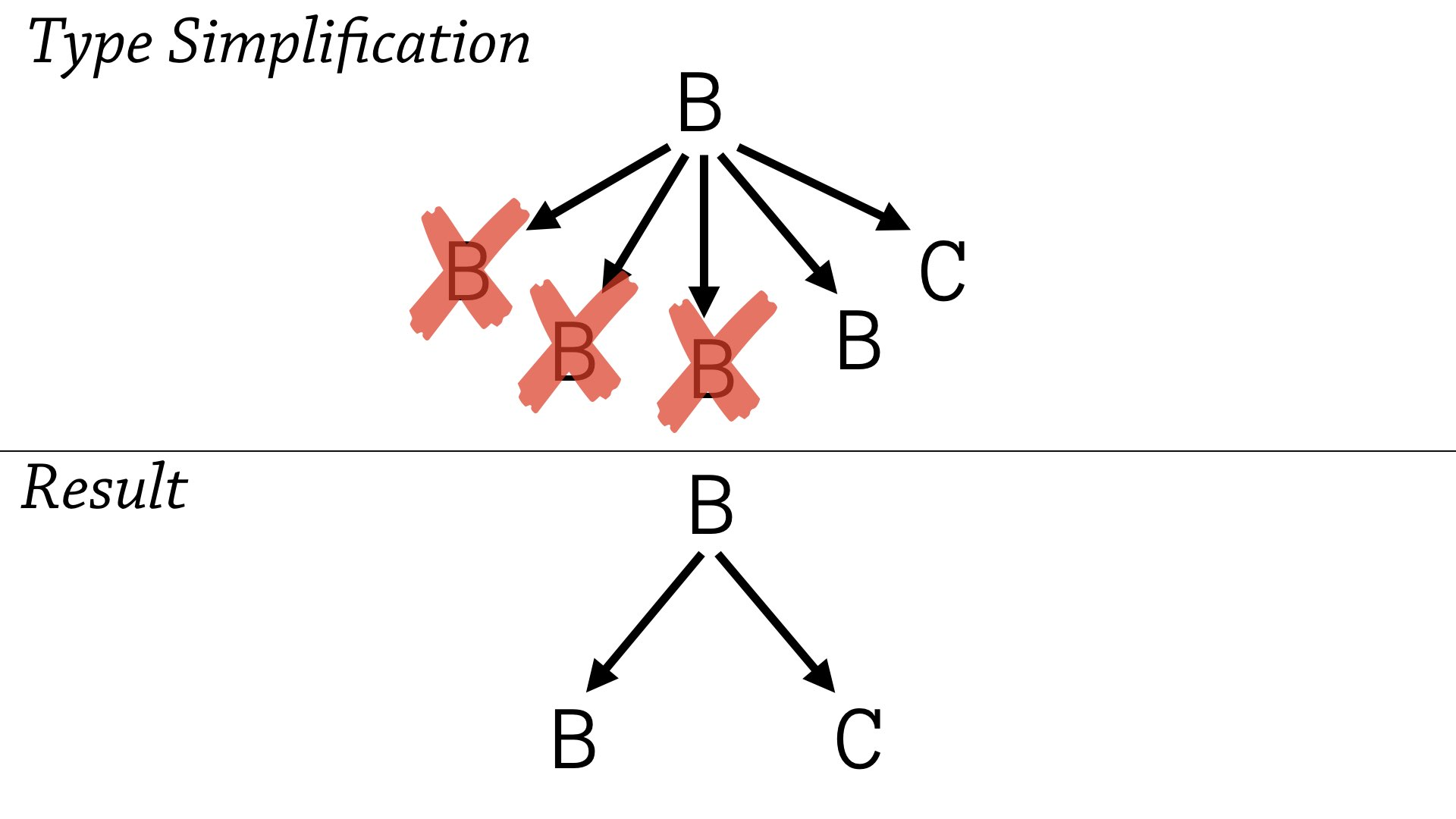# dissoc

## Leaning on a Garbage Collector A Little Too Hard

Our work in automatic annotations for `clojure.spec` uses aliases to create readable, compact, and useful specs. Here’s the kind of thing we’re striving for: informative and pretty alias names (like `Ints` and `Syms`), while reusing aliases more than once (like `Ints` in this example).

``````(s/def Ints (s/coll-of integer?))
(s/def Syms (s/coll-of symbol?))

(s/fdef f
:args (s/cat :x Ints
:y Syms)
:ret Ints)
``````

To achieve this, we start with “naive” spec annotations with no aliases (via runtime observations). Then, we use these specs to create as many aliases as we can. Finally, we merge similar aliases based on several heuristics.

For performance reasons, this merging process always leaves some garbage that is cleaned up in one final sweep. However, our initial approach to merging aliases was subtly flawed and certain situations left so much garbage that we ran out of memory.

# The Problem

Let’s establish a shorthand for aliases that refer to other aliases. Aliases starting with `A` are temporary. Here, `A0`, `A1`, and `A2` are recursive aliases that reference the permanent alias `B`.

``````(s/def A0
(s/coll-of (s/or :a A0
:b B)))
(s/def A1
(s/coll-of (s/or :a A1
:b B)))
(s/def A2
(s/coll-of (s/or :a A2
:b B)))

(s/def B
(s/coll-of C))
``````

We can draw the dependencies of these aliases as a graph, where the edges mean “refers to”. For example, `A0` refers to itself and `B`, so it has edges to `A0` and `B`.Let’s say we’ve decided to merge all these temporary aliases into `B` (see below). First we redirect all the temporary aliases to point directly to `B` (bottom left), then we update `B` to be the combination of all the original aliases (bottom right).The size of `B` is linear in the number of recursive aliases merged into it — that is, `B` gets bigger every time we merge a recursive alias into it. In then end, however, the final garbage collection pass (below) deletes all temporary aliases and results in a much simpler, but redundant, representation for `B` .We can then often simplify these specs to compress all the redundant recursive occurrences of `B` (below). Since we combine specs with unions (called `s/or` in spec), we can use simple set theory to simplify the specs.Now `B` is quite compact! Can we maintain this compact representation throughout the algorithm? This is a crucial question to answer: the size of specs is the main performance bottleneck in the annotation reconstruction algorithm.

# The Solution

The insight here is that most of the aliases `B` refers to are just references to `B` itself. You can see this by tracing through `B` in this example. For example, `B` refers to `A0`, but `A0` just references right back to `B`.

``````(s/def A0 B)
(s/def A1 B)
(s/def A2 B)

(s/def B
(s/or :0 (s/coll-of (s/or :a A0
:b B))
:1 (s/coll-of (s/or :a A1
:b B))
:2 (s/coll-of (s/or :a A2
:b B))
:3 C))
``````

We can tackle this issue by introducing some eager garbage collection. By inlining the new temporary aliases in `B`, we can simplify `B` to:

``````...

(s/def B
(s/or :0 (s/coll-of B)
:1 (s/coll-of B)
:2 (s/coll-of B)
:3 C))
``````

Then, we can erase redundant elements of the union to further compress the spec.

``````(s/def B
(s/or :0 (s/coll-of B)
:1 C))
``````

With this simply policy of eagerly rewriting occurrences of `A0`, `A1`, and `A2` to `B` instead of relying on garbage collection, we gain a critical property to predictable performance: no matter which order we merge the temporary aliases into `B`, we can limit the size of it’s union to 2 members.

# Where does this help?

This linear accumulation of union size is a problem when merging recursive aliases. Fixing the bug enabled us to generate annotations for complicated recursive AST representations, like the ClojureScript compiler’s.

Can we now annotate the ClojureScript compiler’s AST? Not quite, but we’re very close — I’ll end the post with a preview of what our tool now emits after fixing this bug.

``````(defmulti op-multi-spec :op)
(defmethod
op-multi-spec
:constant
[_]
(s/keys :req-un [::env ::form ::op ::tag]))
(defmethod
op-multi-spec
:var
[_]
(s/keys
:req-un
[::env ::info ::op]
:opt-un
[::binding-form?
::column
::form
::init
::line
::local
::name
::tag]))
(defmethod
op-multi-spec
:if
[_]
(s/keys
:req-un
[::children
::else
::env
::form
::op
::tag
::test
::then
::unchecked]))
``````

13 Apr 2018